# How Many Days Left in Month / Year – Excel & Google Sheets

This tutorial will demonstrate how to find the total number of days Remaining in a Month or a Year.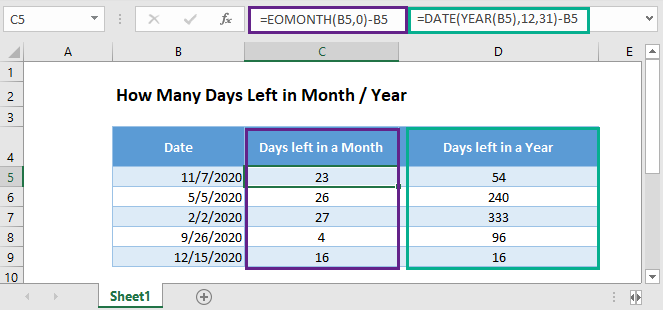## Remaining Days in Month using EOMONTH Function

The EOMONTH Function returns the last day of a month. Its syntax is: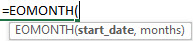We can simply subtract the start date from the last day of the month to find the number of days remaining:

``=EOMONTH(B3,0)-B3``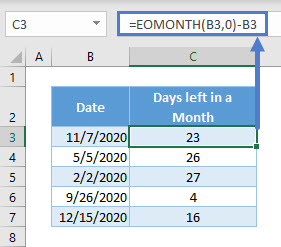## Remaining Days in Month using TODAY Function

This example will calculate the number of days remaining from today.

``=EOMONTH(TODAY(),0)-TODAY()``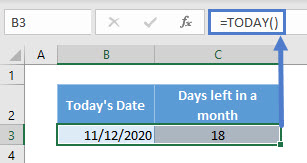The nested TODAY Function returns input for the start_date input for the EOMONTH Function.

## Remaining Days in Year using DATE Function

To calculate the days remaining in a year, we will use the DATE Function. Its syntax is: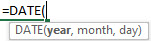Here we will subtract the start date from the last day of the year:

``=DATE(YEAR(B3),12,31)-B3``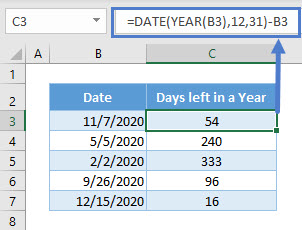The YEAR Function returns the current year. The Date Function outputs the last day of the year.

By subtracting the values we calculate the number of days left in the year.

## Days Remaining in Year using TODAY Function

The following example calculates the remaining number of days in a year starting with today.

``=DATE(YEAR(TODAY()),12,31)-TODAY()``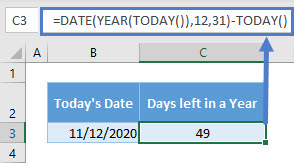## Days Remaining Month/Year in Google Sheets

These formulas work exactly the same in Google Sheets as in Excel.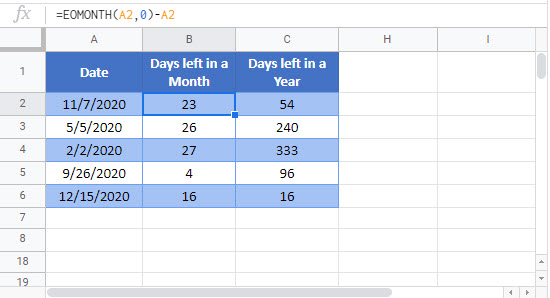### Excel Practice Worksheet

Practice Excel functions and formulas with our 100% free practice worksheets!